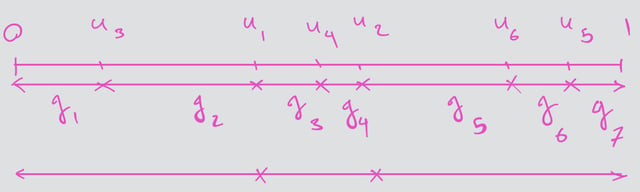# #StackBounty: #bayesian #bootstrap Understanding Bayesian Bootstrap theory

### Bounty: 50

I’m trying to understand the theory in section 4 of Rubin (1981) paper on Bayesian Bootstrap (BB):

$$textbf{Theory:}$$ Let $$d=left(d_{1}, ldots, d_{K}right)$$ be the vector of all possible distinct values of $$X$$, and let $$pi=left(pi_{1}, cdots, pi_{K}right)$$ be the associated vector of probabilities
$$Pleft(X=d_{k} mid piright)=pi_{k}, quad sum pi_{k}=1$$
Let $$x_{1}, ldots, x_{n}$$ be an i.i.d. sample from the equation above and let $$n_{k}$$ be the number of $$x_{i}$$ equal to $$d_{k}$$. If the prior distribution of $$pi$$ is proportional to
$$prod_{k=1}^{K}pi_{k}^{l_k}quad left(0right. text { if } left.sumpi_{k} neq 1right)$$
then the posterior distribution of $$pi$$ is the $$K-1$$ variate Dirichlet distribution $$Dleft(n_{1}+l_{1}+1,right.$$ $$left.ldots, n_{K}+l_{K}+1right)$$ which is proportional to
$$quad prod_{k=1}^{K} pi_{k}^{left(n_{k}+l_{k}right)} quadleft(0right. text{ if } x_{imath} neq d_{k} text{for some } i, k text{ or if} left.sum pi_{k} neq 1right)$$

• What does $$K-1$$ variate mean?

This posterior distribution can be simulated using $$m-1$$ independent uniform random numbers, where $$m=n+K+sum_{1}^{K} l_{k}$$.

• Where does this come from?

Let $$u_{1}, cdots, u_{m-1}$$ be i.i.d. $$U(0,1),$$ and let $$g_{1}, cdots, g_{m}$$ be the $$m$$ gaps generated by the ordered $$u_{imath}$$. Partition the $$g_{1}, cdots, g_{m}$$ into $$K$$ collections, the $$k$$-th having $$n_{k}+l_{k}+1$$ elements,

• Is element referring to $$u$$‘s or gaps? I think gaps because $$sum_1^K(n_{k}+l_{k}+1)=m$$. If so, is partitioning mean to group adjacent gaps together? Something like the bottom line below for $$m=7$$ and $$K=3$$?and let $$P_{k}$$ be the sum of the $$g_{i}$$ in the $$k$$-th collection, $$k=1, cdots, K$$.

• Does this mean $$P_{k}$$ is the size of collection $$k$$? Does "sum of the $$g_{i}$$" mean sum of the length of $$g_{i}$$‘s?

Then $$left(P_{1}, ldots, P_{K}right)$$ follows the $$K-1$$ variate $$Dleft(n_{1}+l_{1}+1, ldots, n_{K}+l_{K}+1right)$$ distribution. Consequently, the BB which assigns one gap to each $$x_{i}$$

• But we have $$m$$ gaps vs. $$n$$ $$x_i$$‘s. How does this work?

is simulating

• What does simulating mean in this context?

the posterior distribution of $$pi$$ and thus of a parameter $$phi=Phi(pi, d)$$ under the improper prior distribution proportional to $$prod_{k=1}^{K} pi_{k}^{-1}$$.

• Where did the $$l_k=-1$$ come from?

Simulations corresponding to other prior distributions with integer $$l_{k}$$ can also be performed; for example, with a uniform prior distribution on $$pi$$, (i.e., all $$l_{k}=0$$ ) generate $$n+K-1$$ uniform random variables, form $$n+K$$ gaps, add the first $$left(n_{1}+1right)$$ gaps together to yield the simulated value of $$pi_{1}$$, add the second $$left(n_{2}+1right)$$ gaps together to yield the simulated value of $$pi_{2}$$, and so on. However, when using a proper prior distribution, all a priori possible values of $$X$$ must be specified because they have positive posterior probability.

• What does "all a priori possible values of $$X$$ must be specified" mean and how is this different from the previous case of improper prior with $$l_k=-1$$?

Get this bounty!!!

This site uses Akismet to reduce spam. Learn how your comment data is processed.